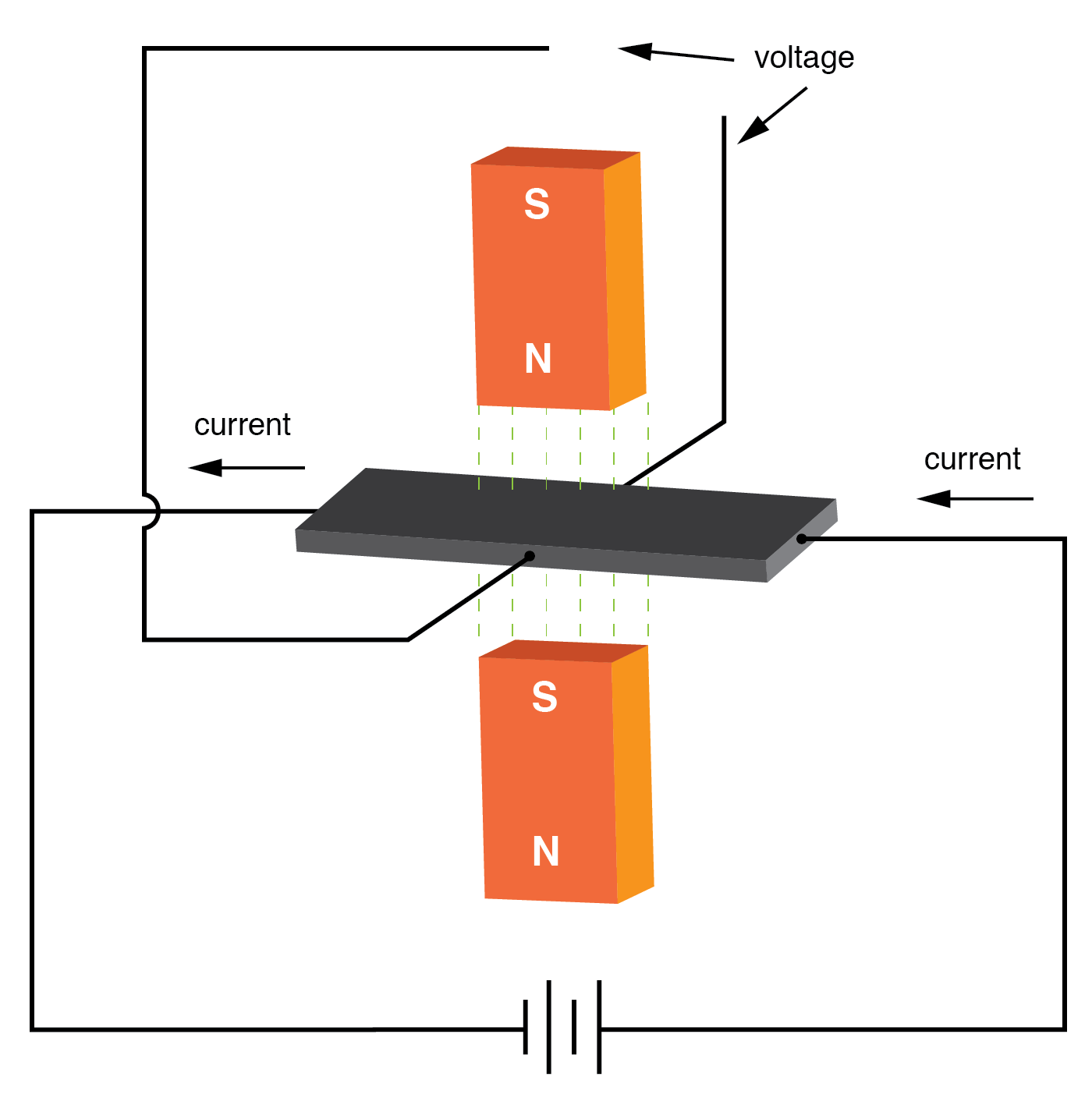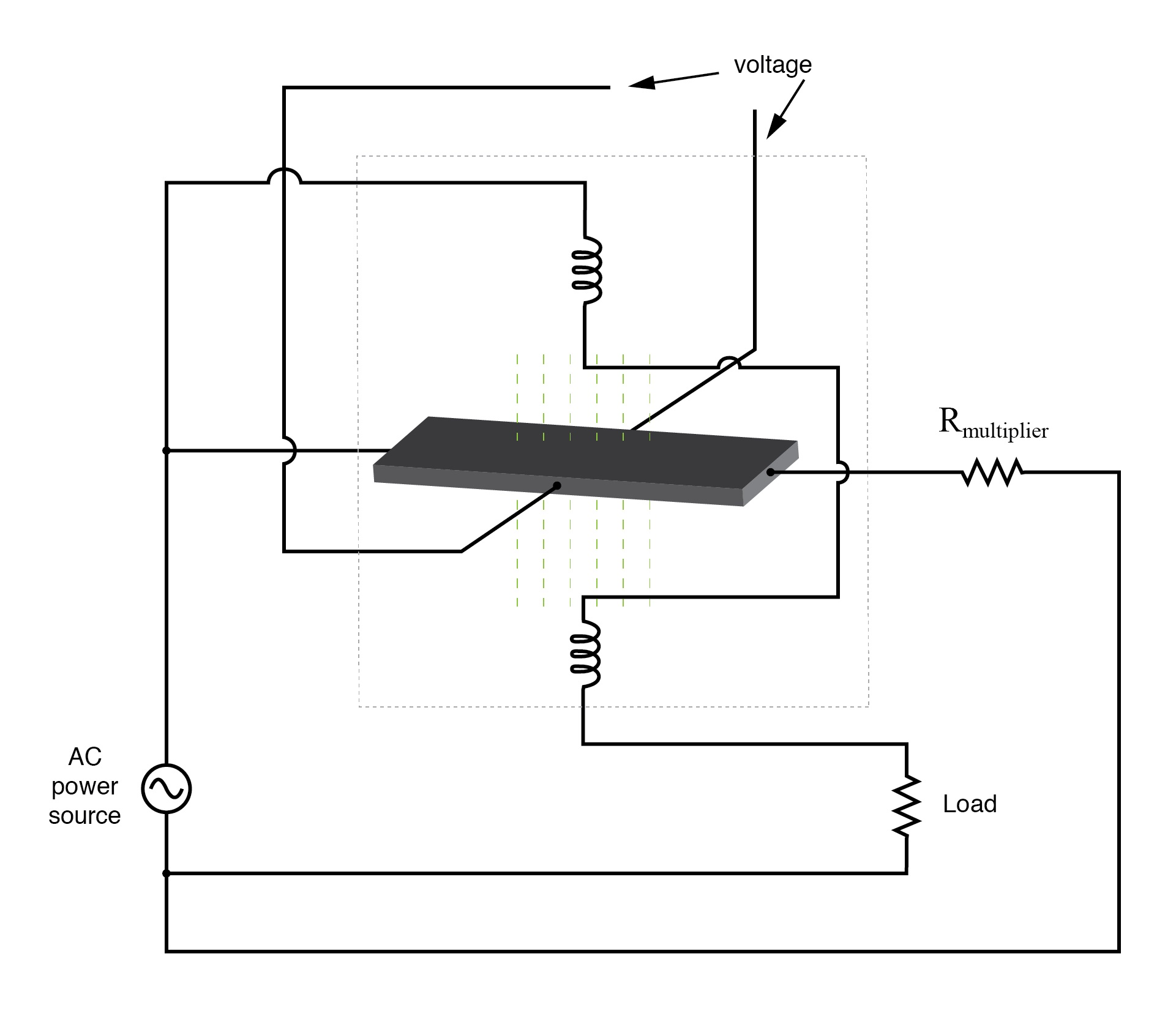# 12.3 Power Measurement

Power measurement in AC circuits can be quite a bit more complex than with DC circuits for the simple reason that phase shift complicates the matter beyond multiplying the voltage by current figures obtained with meters. What is needed is an instrument able to determine the product (multiplication) of instantaneous voltage and current. Fortunately, the common electrodynamometer movement with its stationary and moving coil does a fine job of this.

Three phase power measurement can be accomplished using two dynamometer movements with a common shaft linking the two moving coils together so that a single pointer registers power on a meter movement scale. This, obviously, makes for a rather expensive and complex movement mechanism, but it is a workable solution.

### Hall Effect

An ingenious method of deriving an electronic power meter (one that generates an electric signal representing power in the system rather than merely moves a pointer) is based on the Hall effect. The Hall effect is an unusual effect first noticed by E. H. Hall in 1879, whereby a voltage is generated along the width of a current-carrying conductor exposed to a perpendicular magnetic field:Hall effect: Voltage is proportional to current and strength of the perpendicular magnetic field.

The voltage generated across the width of the flat, rectangular conductor is directly proportional to both the magnitude of the current through it and the strength of the magnetic field. Mathematically, it is a product (multiplication) of these two variables. The amount of “Hall Voltage” produced for any given set of conditions also depends on the type of material used for the flat, rectangular conductor. It has been found that specially prepared “semiconductor” materials produce a greater Hall voltage than do metals, and so modern Hall Effect devices are made of these.

It makes sense then that if we were to build a device using a Hall-effect sensor where the current through the conductor was pushed by AC voltage from an external circuit and the magnetic field was set up by a pair of wire coils energized by the current of the AC power circuit, the Hall voltage would be in direct proportion to the multiple of circuit current and voltage. Having no mass to move (unlike an electromechanical movement), this device is able to provide instantaneous power measurement:Hall effect power sensor measures instantaneous power.

Not only will the output voltage of the Hall effect device be the representation of instantaneous power at any point in time, but it will also be a DC signal! This is because the Hall voltage polarity is dependent upon both the polarity of the magnetic field and the direction of current through the conductor. If both current direction and magnetic field polarity reverse—as it would ever half-cycle of the AC power—the output voltage polarity will stay the same.

If voltage and current in the power circuit are 90° out of phase (a power factor of zero, meaning no real power delivered to the load), the alternate peaks of Hall device current and magnetic field will never coincide with each other: when one is at its peak, the other will be zero. At those points in time, the Hall output voltage will likewise be zero, being the product (multiplication) of current and magnetic field strength. Between those points in time, the Hall output voltage will fluctuate equally between positive and negative, generating a signal corresponding to the instantaneous absorption and release of power through the reactive load. The net DC output voltage will be zero, indicating zero true power in the circuit.

Any phase shift between voltage and current in the power circuit less than 90° will result in a Hall output voltage that oscillates between positive and negative but spends more time positive than negative. Consequently, there will be a net DC output voltage. Conditioned through a low-pass filter circuit, this net DC voltage can be separated from the AC mixed with it, the final output signal registered on a sensitive DC meter movement.

Often it is useful to have a meter to totalize power usage over a period of time rather than instantaneously. The output of such a meter can be set in units of Joules, or total energy consumed since power is a measure of work being done per unit time. Or, more commonly, the output of the meter can be set in units of Watt-Hours.

Back to Main Index of Book